天线调试匹配方法

2018-04-12 来源：百度文库 我要评论(0) 字号：

“仿真工具在实际工程中没有什么用处”，是说在设计匹配电路时，更具体一点是指设计双频GSM、DCS手机天线匹配电路时。如果单独理解这句话，无疑是错的。事实上，我一直在用HFSS进行天线仿真，其结果也都是基于仿真结果的。

·   计算机仿真： 由于这类软件是为不同功能设计的而不只是用于阻抗匹配，所以使用起来比较复杂。设计者必须熟悉用正确的格式输入众多的数据。设计人员还需要具有从大量的输出结果中找到有用数据的技能。另外，除非计算机是专门为这个用途制造的，否则电路仿真软件不可能预装在计算机上。

·   手工计算： 这是一种极其繁琐的方法，因为需要用到较长(“几公里”)的计算公式、并且被处理的数据多为复数。

·   经验： 只有在RF领域工作过多年的人才能使用这种方法。总之，它只适合于资深的专家。

·   史密斯圆图：本文要重点讨论的内容。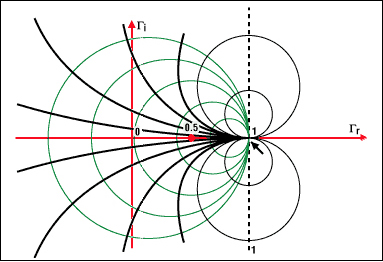RS + jXS = RL - jXL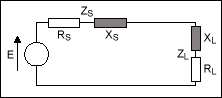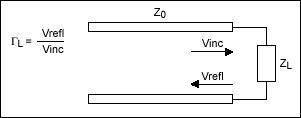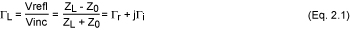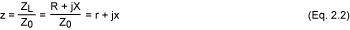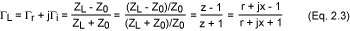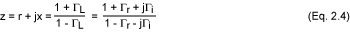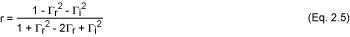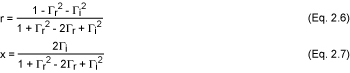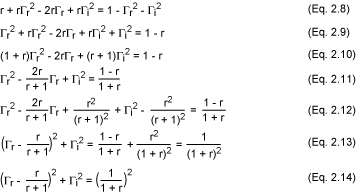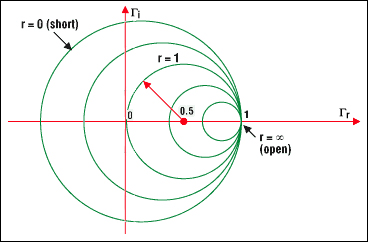·   所有的圆周只有一个相同的，唯一的交点(1，0)。
·   代表0Ω、也就是没有电阻(r = 0)的圆是最大的圆。
·   无限大的电阻对应的圆退化为一个点(1，0)
·   实际中没有负的电阻，如果出现负阻值，有可能产生振荡。
·   选择一个对应于新电阻值的圆周就等于选择了一个新的电阻。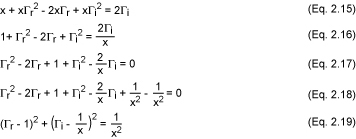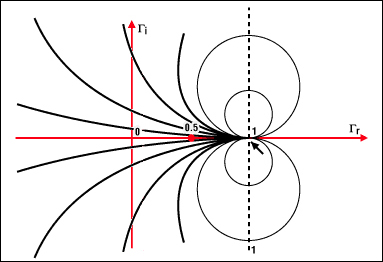·   确定阻抗在史密斯圆图上的对应点
·   找到与此阻抗对应的反射系数(Γ)
·   已知特性阻抗和Γ，找出阻抗
·   将阻抗转换为导纳
·   找出等效的阻抗
·   找出与反射系数对应的元件值(尤其是匹配网络的元件，见7)

 Z1 = 100 + j50Ω Z2 = 75 - j100Ω Z3 = j200Ω Z4 = 150Ω Z5 = ∞ (an open circuit) Z6 = 0 (a short circuit) Z7 = 50Ω Z8 = 184 - j900Ω

 z1 = 2 + j z2 = 1.5 - j2 z3 = j4 z4 = 3 z5 = 8 z6 = 0 z7 = 1 z8 = 3.68 - j18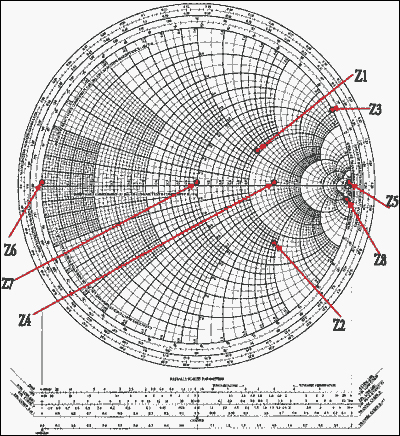Γ1 = 0.4 + 0.2j Γ2 = 0.51 - 0.4j Γ3 = 0.875 + 0.48j Γ4 = 0.5 Γ5 = 1 Γ6 = -1 Γ7 = 0 Γ8 = 0.96 - 0.1j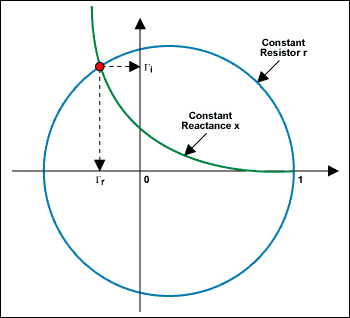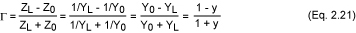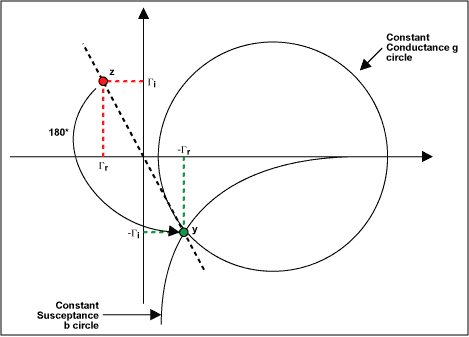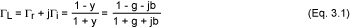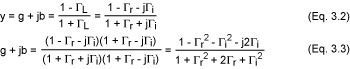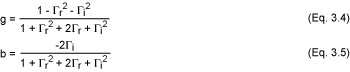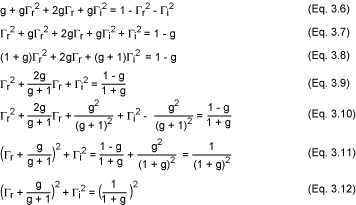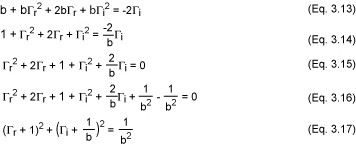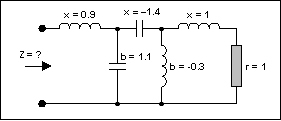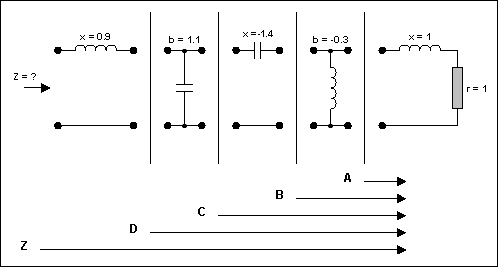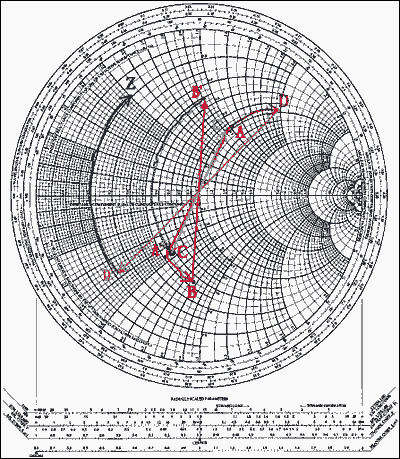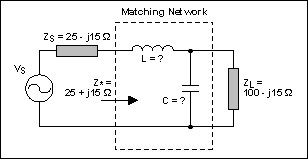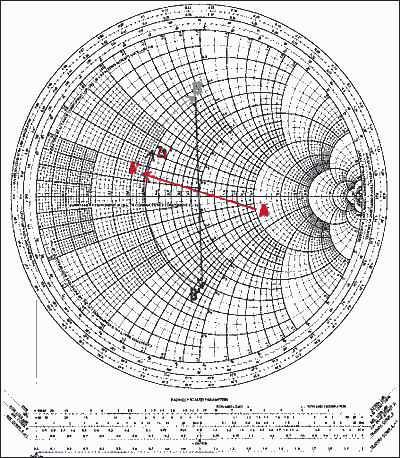B到D的弧长为× = 1.2，于是X = 1.2 × Z0 = 60Ω。由ωL = X，得L = X/ω = X/(2πf)= 60/[2π(60 × 106)] = 159nH。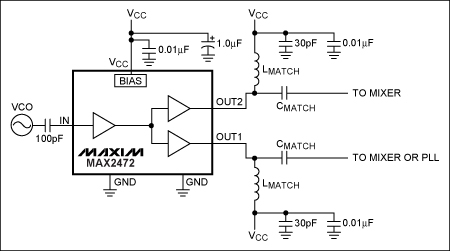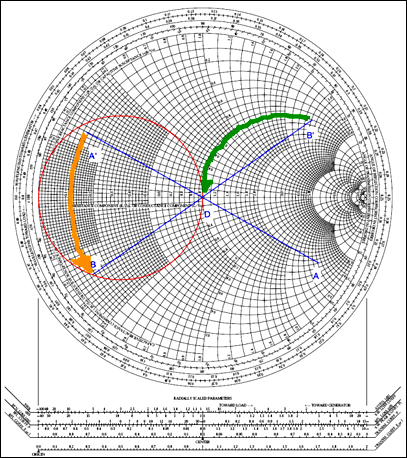微波射频行业人士 | 相聚在这里【10大细分领域的微信技术交流群】订阅微信公众号加群管理员为好友